+91-22-62437100 / 01 | help@reachivy.com

# GMAT Data Sufficiency Quiz 2

Want to strengthen your performance on Data Sufficiency part of the GMAT? Here is a quick test to build your strengths. Test now!

Instructions: This data sufficiency problem consists of a question and two statements, labeled (1) and (2), in which certain data are given. You have to decide whether the data given in the statements are sufficient for answering the question. Using the data given in the statements, plus your knowledge of mathematics and everyday facts (such as the number of days in July or the meaning of the word counterclockwise), you must indicate whether:

Statement (1) ALONE is sufficient, but statement (2) alone is not sufficient to answer the question asked.
Statement (2) ALONE is sufficient, but statement (1) alone is not sufficient to answer the question asked.
BOTH statements (1) and (2) TOGETHER are sufficient to answer the question asked, but NEITHER statement ALONE is sufficient to answer the question asked.
Statements (1) and (2) TOGETHER are NOT sufficient to answer the question asked, and additional data specific to the problem are needed.

Welcome to our GMAT Data Sufficiency Quiz 2. To begin click Next

Question 1:

33 out of the 47 students in an advanced degree program have a higher than average GPA. How many students in the program are receiving some form of academic scholarship?
(1) More students do not have a scholarship than have a scholarship.
(2) The same number of students have a higher than average GPA and are receiving some form of academic scholarship as have neither a higher than average GPA nor an academic scholarship.

Question 2:

Of the Baskin Robbins ice cream stores in the county, 40 stock Rocky Road ice cream, 50 stock Pistachio ice cream, and 35 stock Jamocha ice cream. If each store stocks at least one of these flavors, how many Baskin Robbins stores are in the county?
(1) 42 of the stores stock exactly two of these flavors.
(2) None of the stores stock all three flavors.

Question 3:
A gold cube is melted down and formed into a right cylindrical solid. Is the height of the cylinder greater than twice the height of the cube?
(1) Twice the square of the height of the cube is greater than the circumference of the cylinder.
(2) The height of the cube is equal to the radius of the cylinder.

Question 4:

If x, y, and z ≠ 0, does x ⏀ (y + z) = (x ⏀ y) + (x ⏀ z)?
(1) The symbol ⏀ stands for subtraction
(2) For at least one possible value of x, x⏀1 ≠ 1⏀x

Question 5:

Of the 210 college students who took the two-part Organic Chemistry final, how many did not pass either the multiple-choice test or the written test?
(1) 50% of the test-takers passed the written test.
(2) 60 of the 210 college students passed both sections of the final.

Question 6: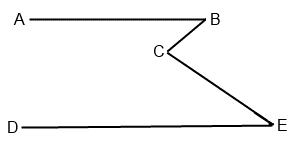Is angle BCE > angle ABC?
(1) AB II DE
(2) Angle CED = 45

Question 7: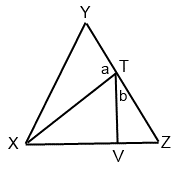If angle TXV = 40, what is the value of the sum of angles a and b?
(1) Triangle XYZ is equilateral.
(2) Triangle TVX is a right triangle.

Question 8: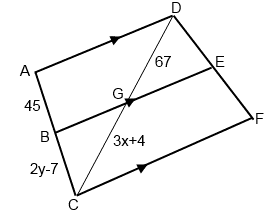What is the ratio of x to y?
(1) DE = EF
(2) y > x
Question 9: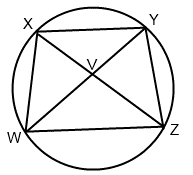What is the value of angle WVZ?
(1) Arc YZ = 78
(2) XY II WZ
Question 10: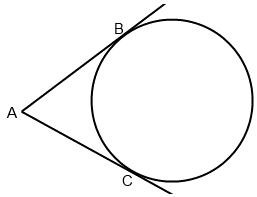What are the values of minor arc and major arc BC?
(1) The difference between the major arc BC and minor arc BC is 124.
(2) Angle BAC = 62

You have answered all the questions in the quiz. Press Submit to view your score